### Home > MC2 > Chapter 7 > Lesson 7.2.3 > Problem7-88

7-88.
1. Simplify each expression below. Homework Help ✎

1.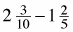2.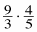3.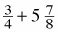4.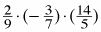5.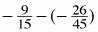6.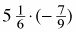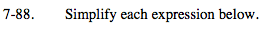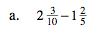Simplifying the whole numbers and fractions separately may make this expression easier to solve.

$(2 - 1) + \left( \frac{3}{5} - \frac{2}{5} \right)$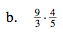Multiply the numerators together and the denominators together.

$\frac{9(4)}{3(5)}$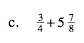See (a).

$-\frac{4}{15}$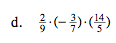See (b).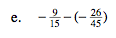Find a common denominator and simplify.

$-\frac{27}{45} - \left(-\frac{26}{45}\right)$

$-\frac{1}{45}$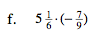Converting the mixed number into a fraction greater than one may make this expression easier to solve.

$-3 \frac{4}{9}$